# Plotting the DTFT using the output of fft

In my Fourier transform series I've been trying to address some of the common points of confusion surrounding this topic. For today's espisode I want to look at how to use the fft function to produce discrete-time Fourier transform (DTFT) magnitude plots in the form you might see in a textbook. Recall that the fft computes the discrete Fourier transform (DFT). I described the relationship between the DFT and the DTFT in my March 15 post.

For my example I'll work with a sequence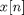that equals 1 for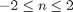and equals 0 elsewhere.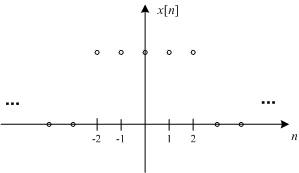Here's a plot of the DTFT magnitude of this sequence: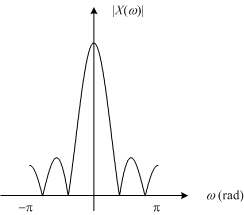Now let's see what get using fft.

x = ones(1, 5)
x =

1     1     1     1     1


X = fft(x);
plot(abs(X))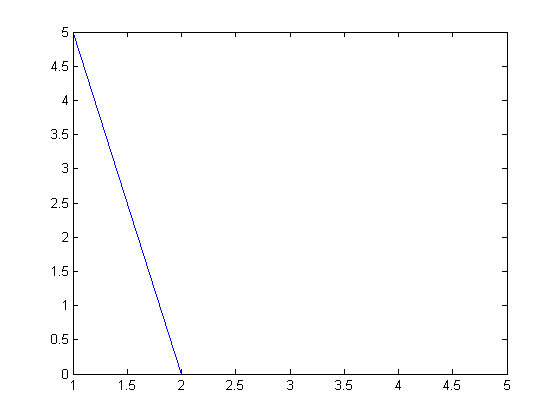Wow, that's not anywhere close to the DTFT magnitude plot above. And why does it look like it's only got two points? Well, take a look at the actual values of X:

X
X =

5     0     0     0     0



We have a 5 and four 0s. What's going on? I explained this back in my March 15 post when I discussed the relationship between the DFT and the DTFT. The outputs of the DFT are samples of the DTFT, and in this case the sample locations just happen to align with the locations of four zeros in the DTFT.

You can get a finer sampling (and a much nicer-looking DTFT plot) by zero-padding. Here I'll use the zero-padding syntax of fft.

N = 256;
X = fft(x, N);
plot(abs(X))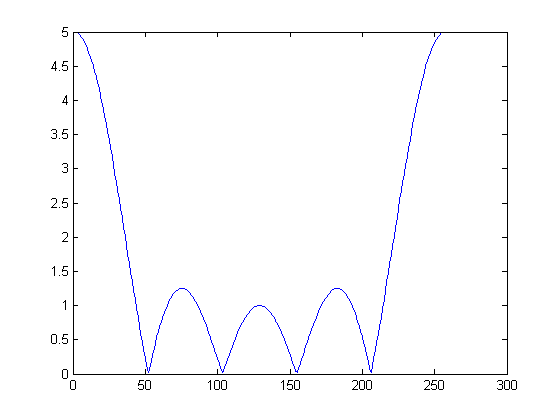That's a smoother-looking curve, but it still looks quite a bit different than the DTFT magnitude plot above. To explain the MATLAB output we're looking at, let me show a DTFT magnitude plot that shows three periods instead of just one.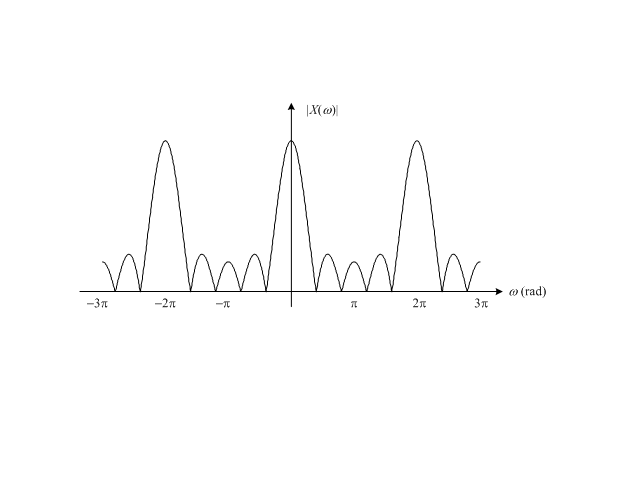You can see that the output from MATLAB is one period of the DTFT, but it's not the period normally plotted, which is fromto. Instead, it's the period from 0 to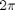. To get a plot fromto, use the fftshift function.

plot(abs(fftshift(X)))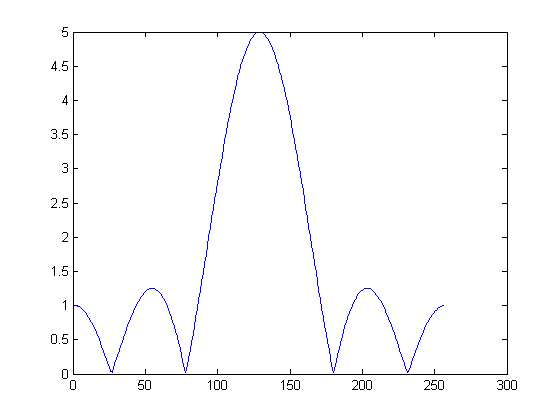That leaves us with the question of labeling the frequency axis. We want a plot in radians fromto.

The way I always remember the frequency scaling between the DFT and the DTFT is this: the length of the DFT corresponds to the frequencyin the DTFT.

So the frequencies in radians corresponding to the output elements of fft are:

w = 2*pi * (0:(N-1)) / N;

But we're calling fftshift to plot the magnitude of the DTFT, so we have to perform a similar shift on our frequencies:

w2 = fftshift(w);
plot(w2)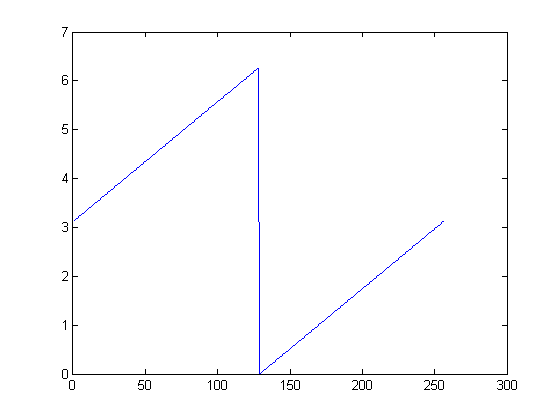Now our frequencies start atand have adiscontinuity in the middle. Here's one way to fix that up:

w3 = unwrap(w2 - 2*pi);
plot(w3)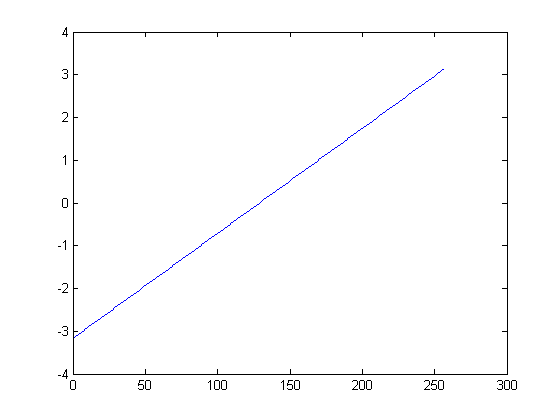Now we can redo our magnitude DTFT plot with the x-axis labels.

plot(w3, abs(fftshift(X)))
xlabel('radians')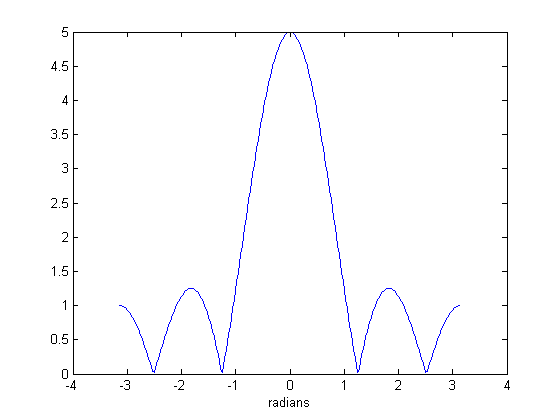Often I like to see the multiples ofmore clearly along the x-axis. One way to accomplish this is to normalize the frequency variable by.

plot(w3/pi, abs(fftshift(X)))
xlabel('radians / \pi')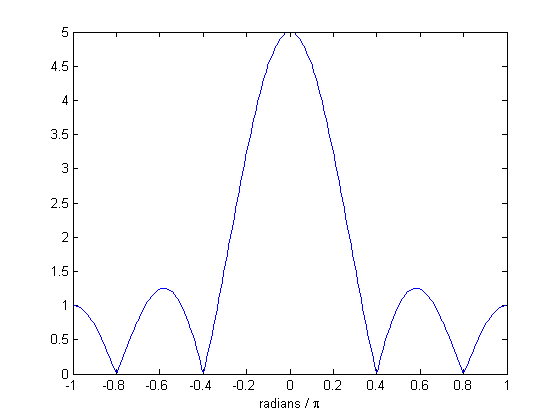Another option is to try Alan's pilabels contribution on the MATLAB Central File Exchange.

For the next time, I'm thinking of tackling the question of why the following output is complex:

fft([1 2 3 2 1])
ans =

9.0000            -2.1180 - 1.5388i   0.1180 + 0.3633i   0.1180 - 0.3633i  -2.1180 + 1.5388i



Many people expect it to be real.

Published with MATLAB® 7.10

|